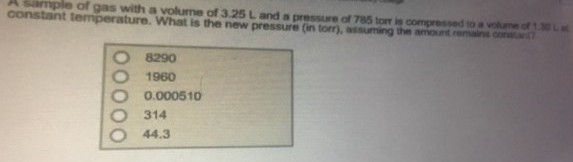# Solution: A sample of gas with a volume of 3.25 L and a pressure of 785 torr is compressed to a volume of 1.30 L at constant temperature. What is the new pressure (in torr), assuming the amount remains constant?a. 8290 b. 1960 c. 0.000510 d. 314e. 44.3

###### Problem

A sample of gas with a volume of 3.25 L and a pressure of 785 torr is compressed to a volume of 1.30 L at constant temperature. What is the new pressure (in torr), assuming the amount remains constant?

a. 8290

b. 1960

c. 0.000510

d. 314

e. 44.3View Complete Written Solution